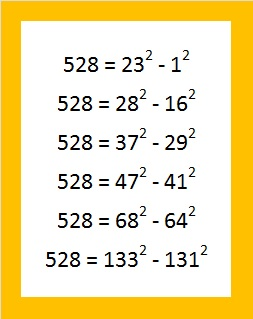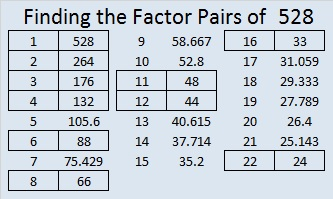# 528 and Level 6

528 feet is one tenth of a mile. Joseph Nebus has written a fascinating post on WHY a mile is 5280 feet. Factoring that number is part of the reason.

Since 528 = (32 x 33)/ 2, we know that 528 is the 32nd triangular number.

Thus, 1 + 2 + 3 + 4 + . . . . . + 29 + 30 + 31 + 32 = 528.

528 can be written as the difference of two squares six different ways:How did I find them? Choose a factor pair where both factors are even or both factors are odd. Average the two factors and that will be the first number to be squared. Square it and subtract 528. Take the square root of the result. That number is the second number in the difference of two squares.Print the puzzles or type the solution on this excel file: 12 Factors 2015-06-15

—————————————————————————————————

• 528 is a composite number.
• Prime factorization: 528 = 2 x 2 x 2 x 2 x 3 x 11, which can be written 528 = (2^4) x 3 x 11
• The exponents in the prime factorization are 4, 1 and 1. Adding one to each and multiplying we get (4 + 1)(1 + 1)(1 + 1) = 5 x 2 x 2 = 20. Therefore 528 has exactly 20 factors.
• Factors of 528: 1, 2, 3, 4, 6, 8, 11, 12, 16, 22, 24, 33, 44, 48, 66, 88, 132, 176, 264, 528
• Factor pairs: 528 = 1 x 528, 2 x 264, 3 x 176, 4 x 132, 6 x 88, 8 x 66, 11 x 48, 12 x 44, 16 x 33 or 22 x 24
• Taking the factor pair with the largest square number factor, we get √528 = (√16)(√33) = 4√33 ≈ 22.97825—————————————————————————————————This site uses Akismet to reduce spam. Learn how your comment data is processed.